# RD Sharma Solutions for Class 8 Maths Chapter 14 Compound Interest Exercise 14.2

As we have seen in the previous exercise, the method of computing compound interest was very lengthy and requires more time to solve. In Exercise 14.2 of Chapter 14, we shall discuss some formulae for the computation of compound interest, which becomes very easy to solve and less time-consuming. Students can access the RD Sharma Solutions which is formulated by our expert faculty team with cares to help students prepare for their exams without any hassle. Students can refer and download the pdf from the links given below.

## Download the Pdf of RD Sharma Solutions for Class 8 Maths Exercise 14.2 Chapter 14 Compound Interest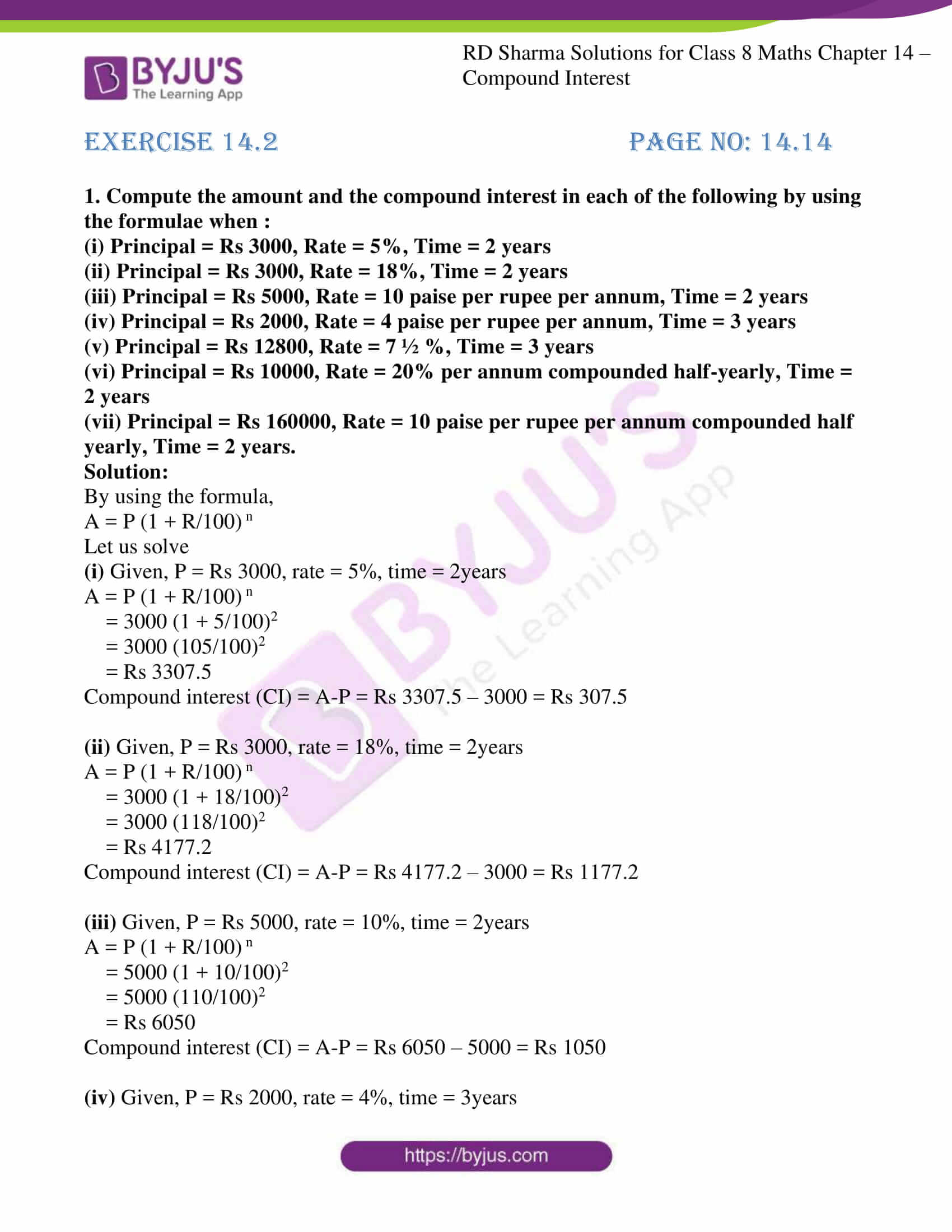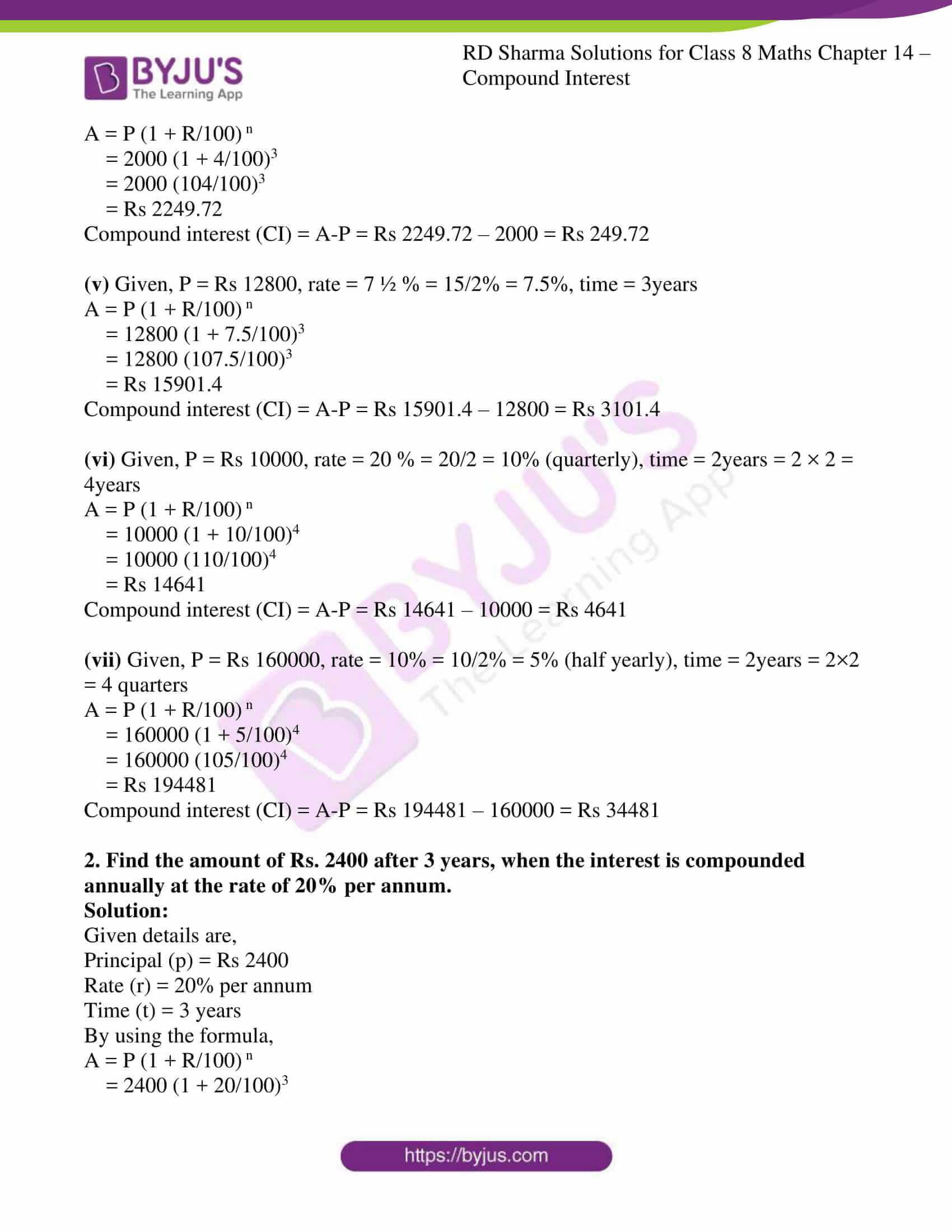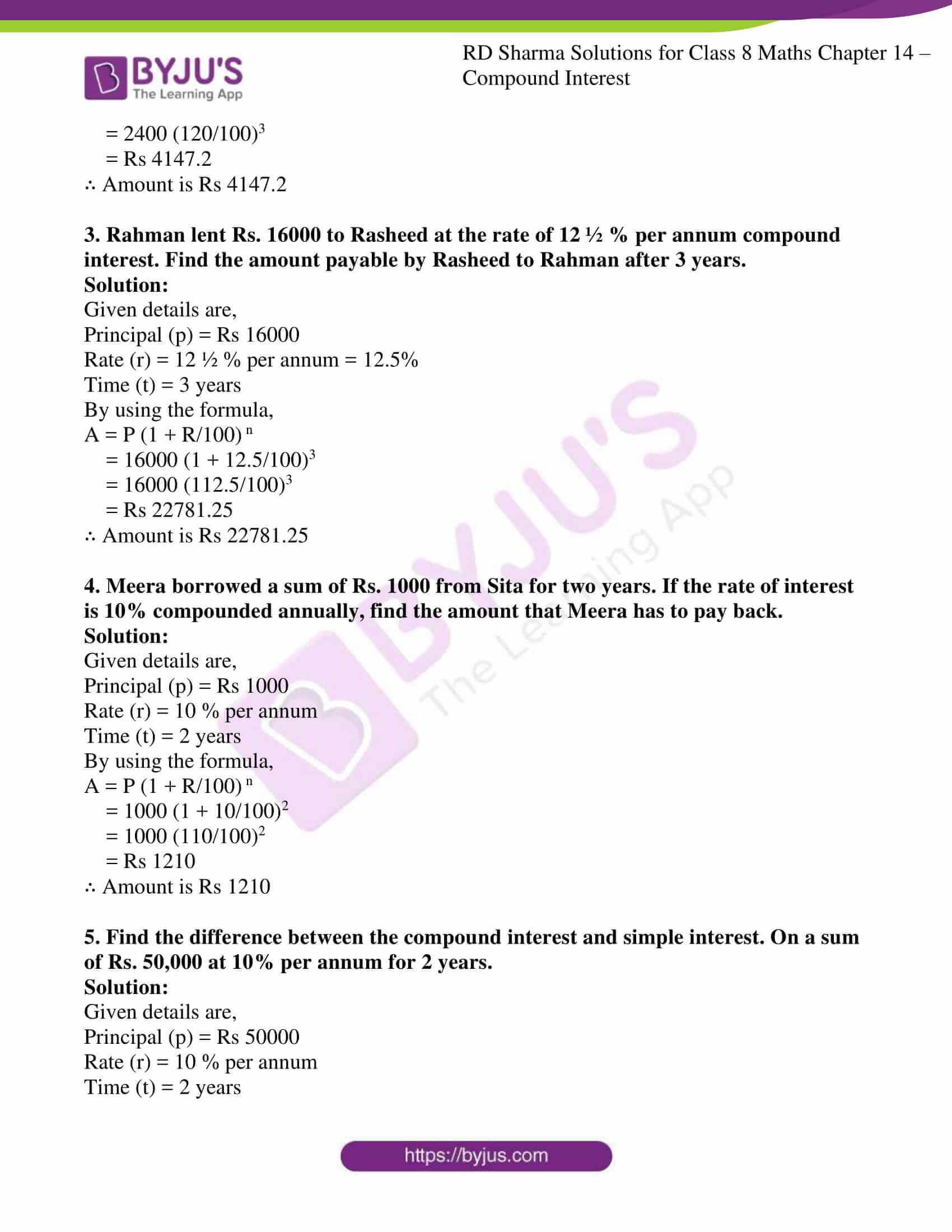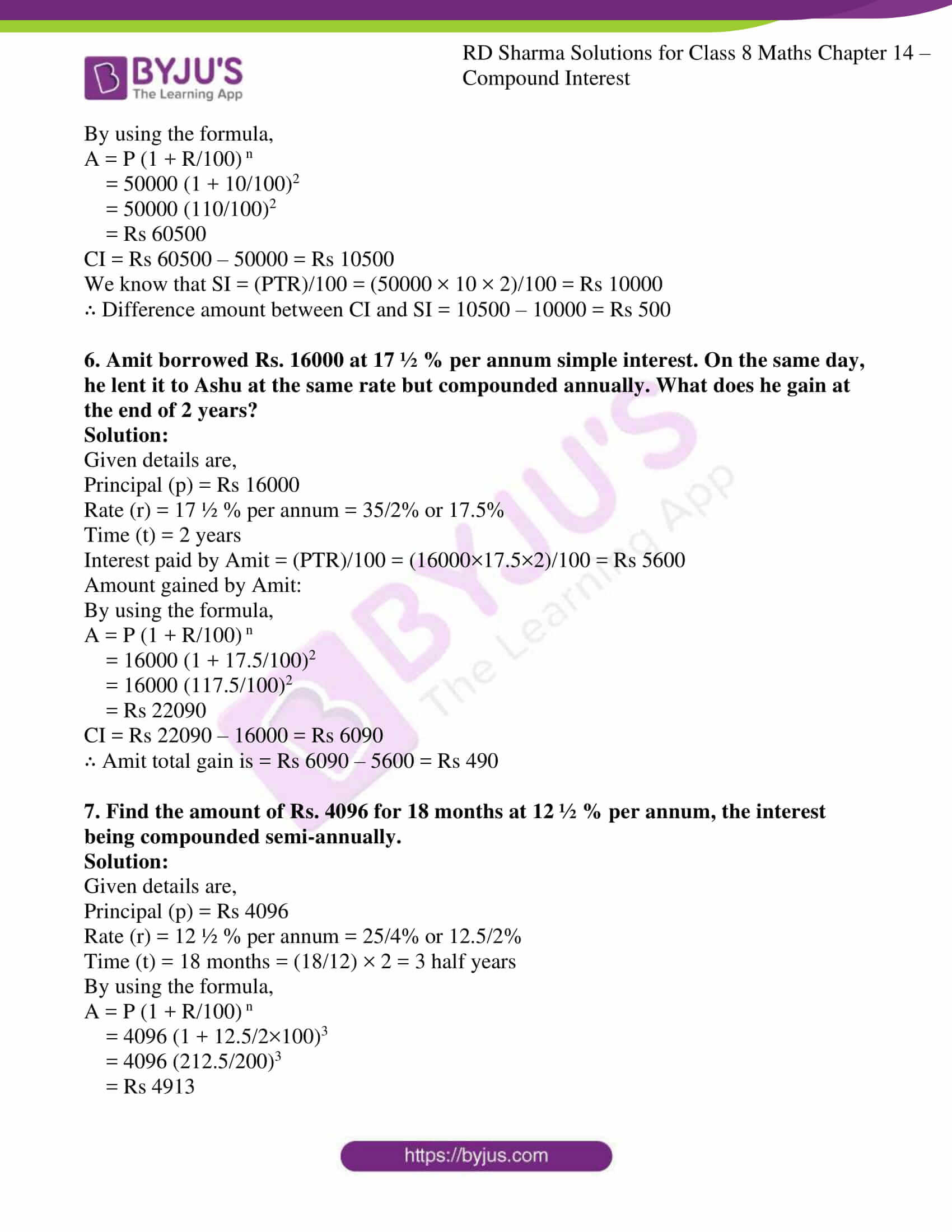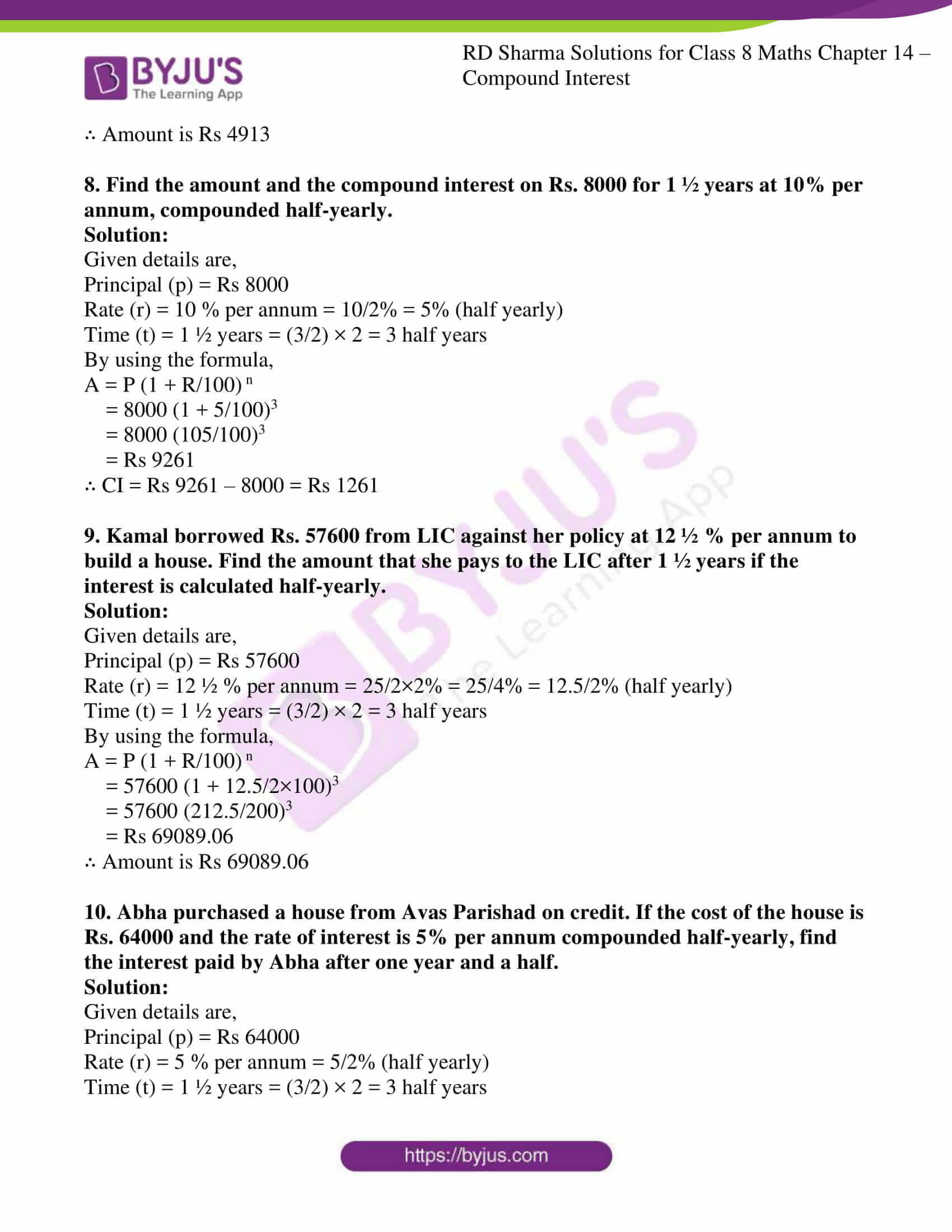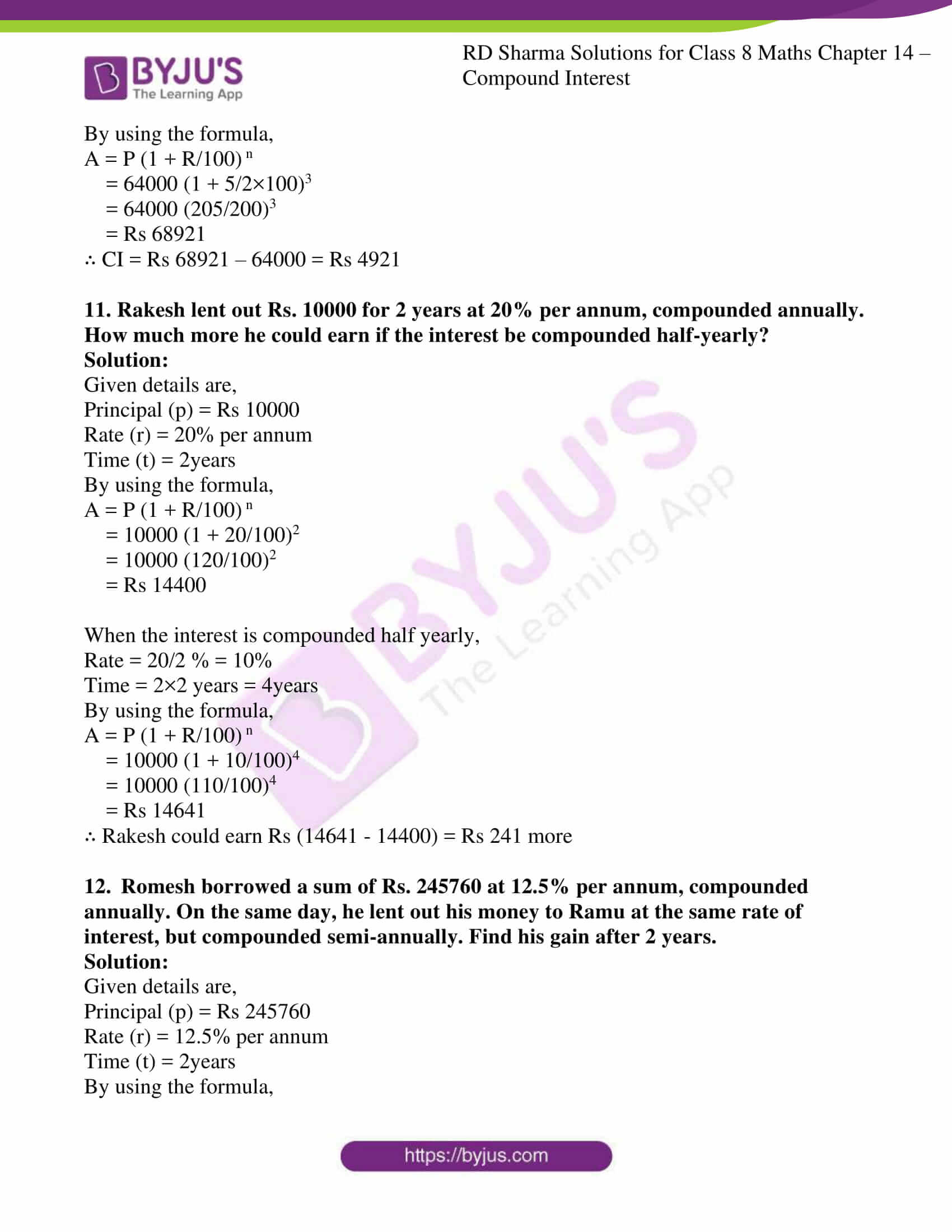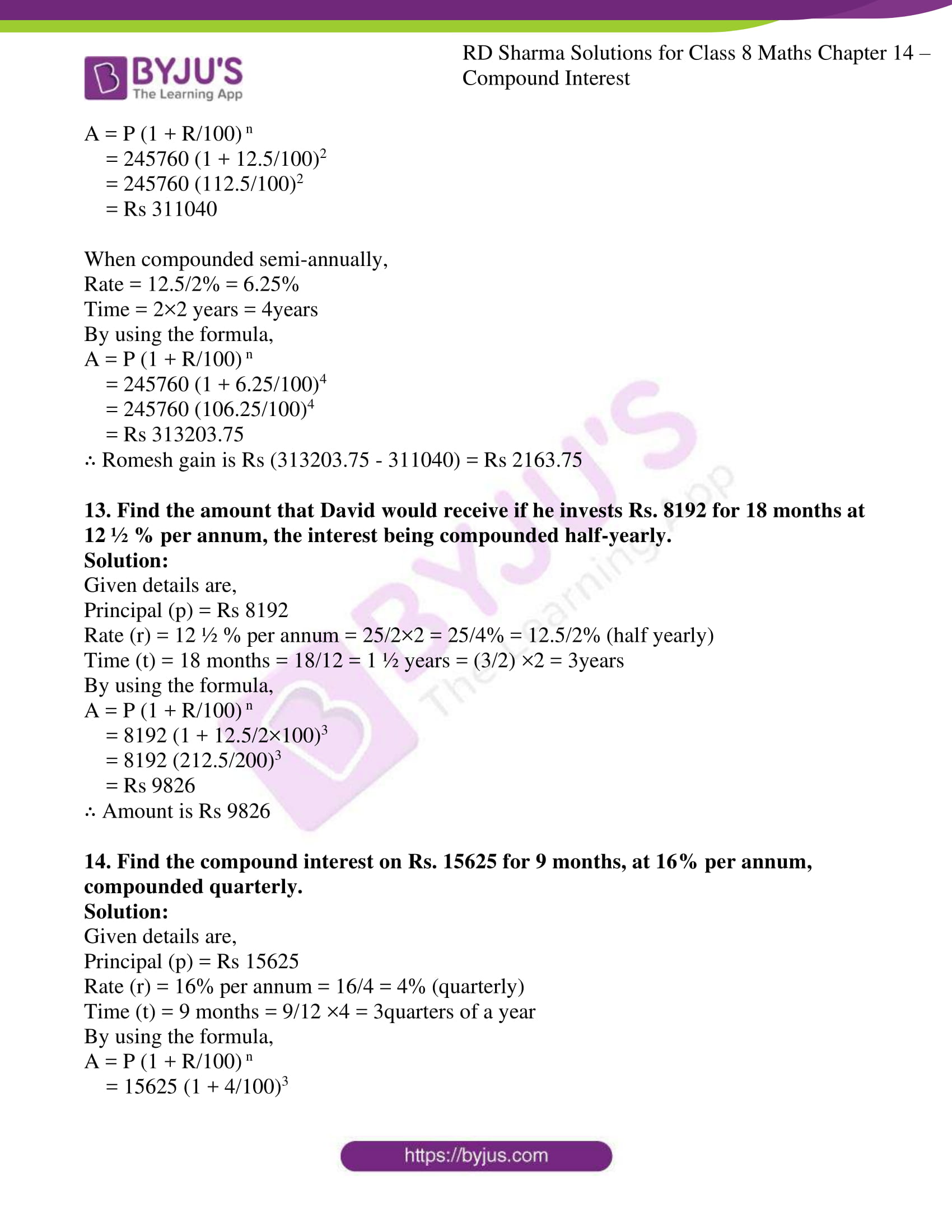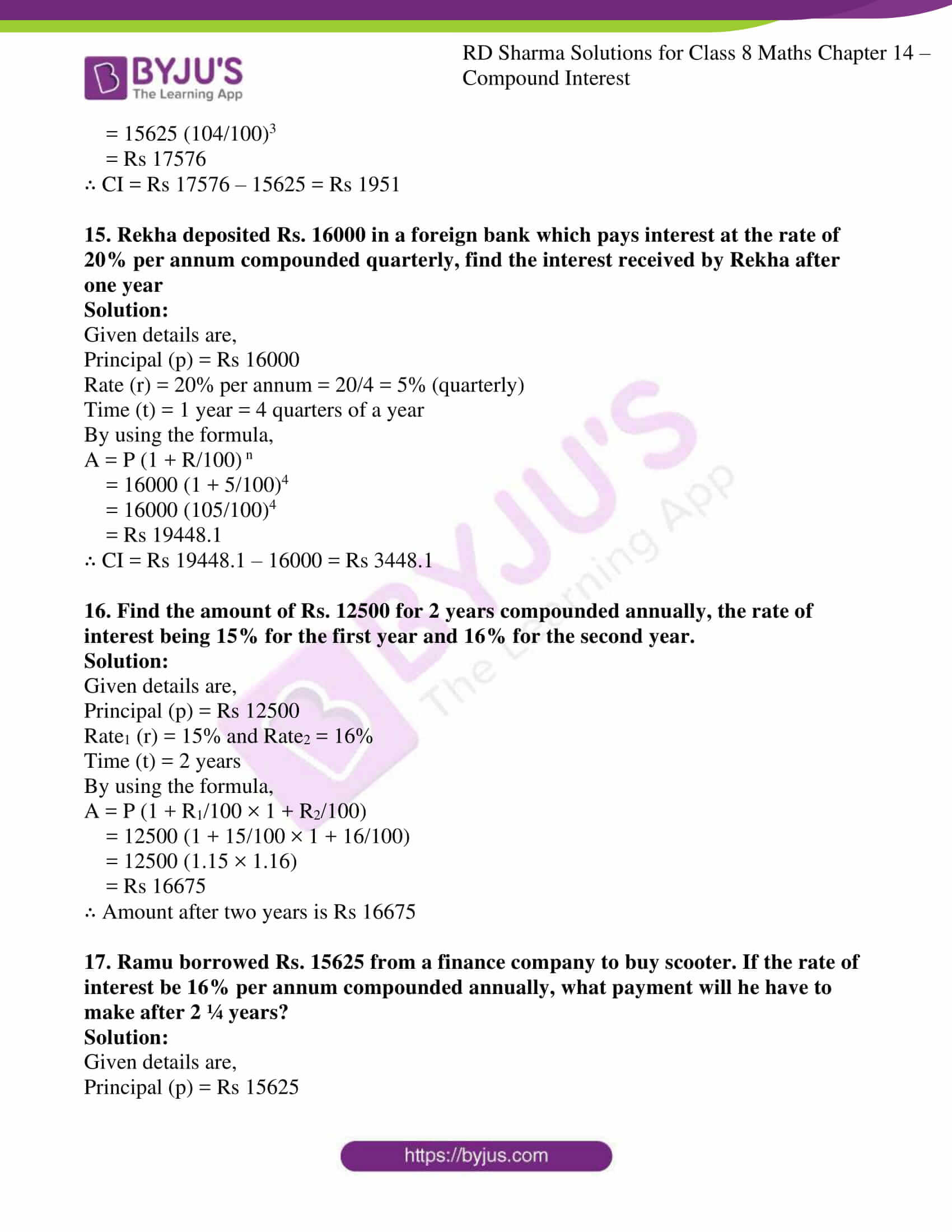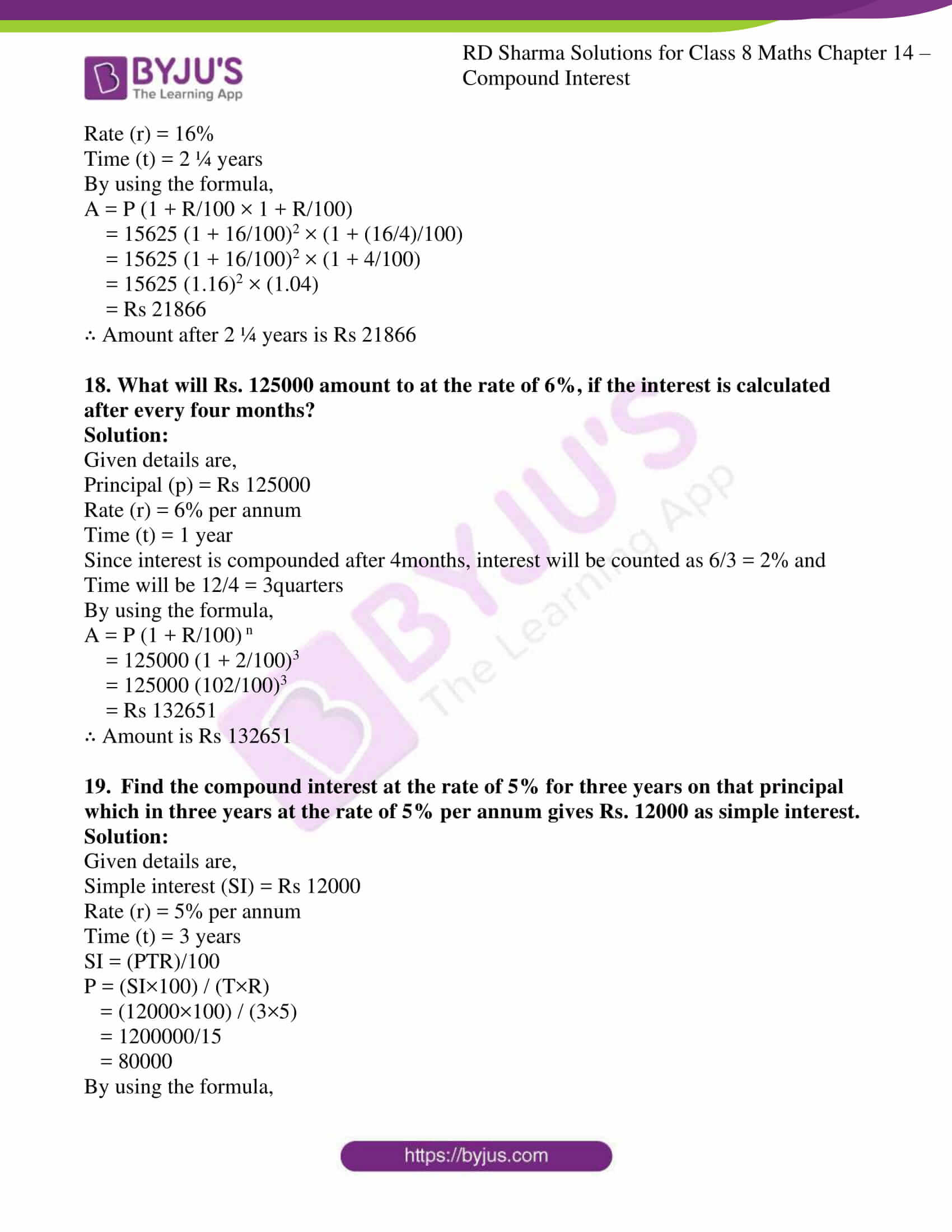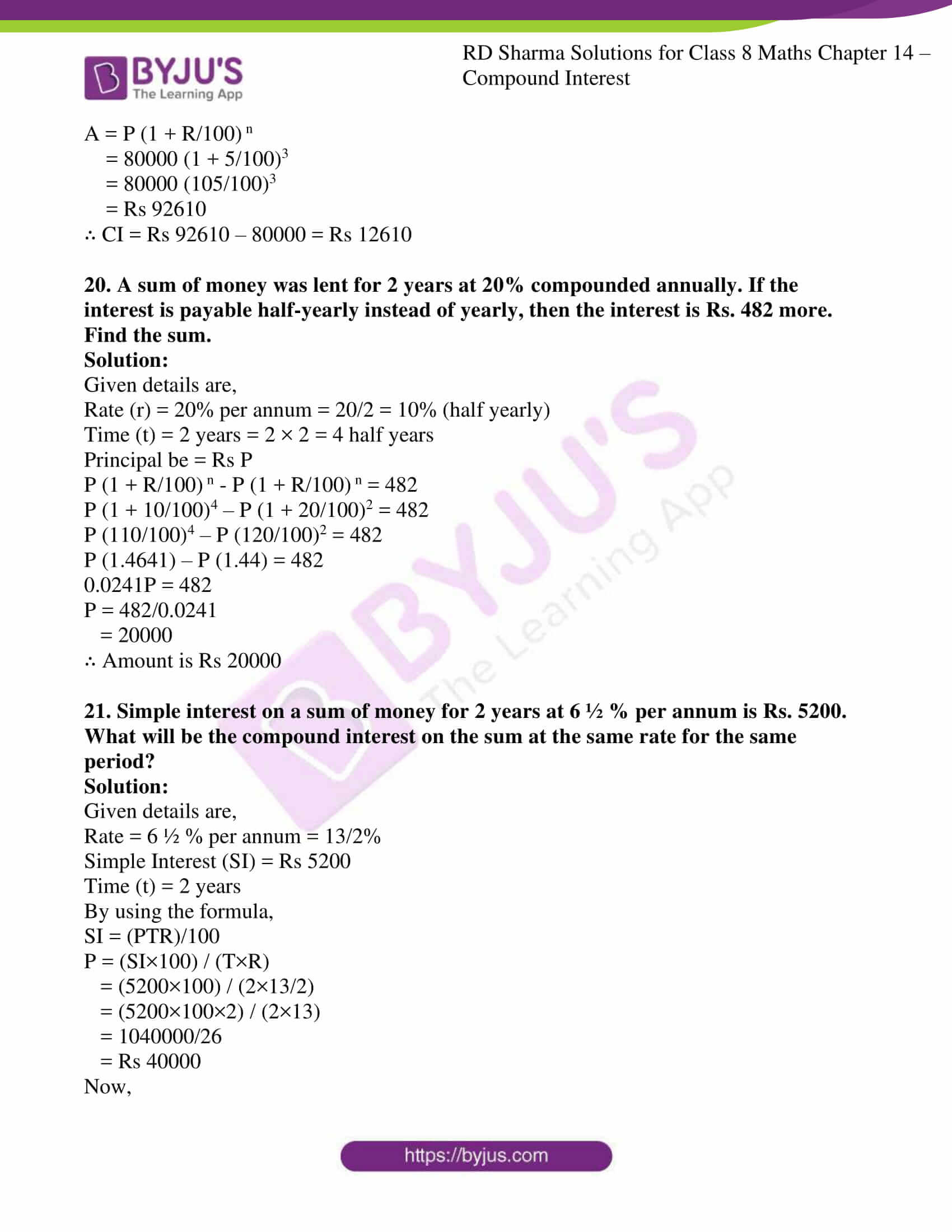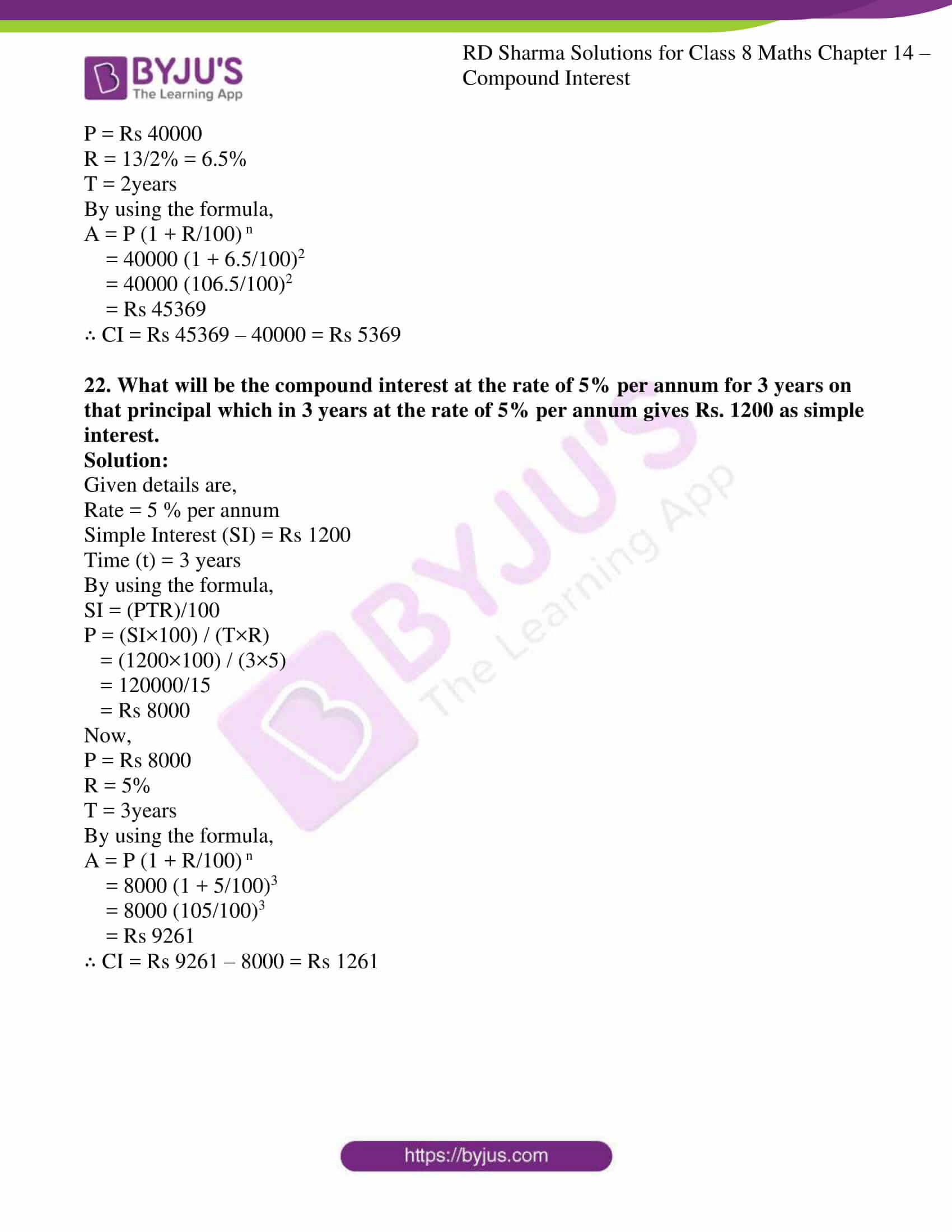### Access answers to Maths RD Sharma Solutions For Class 8 Exercise 14.2 Chapter 14 Compound Interest

1. Compute the amount and the compound interest in each of the following by using the formulae when :
(i) Principal = Rs 3000, Rate = 5%, Time = 2 years
(ii) Principal = Rs 3000, Rate = 18%, Time = 2 years
(iii) Principal = Rs 5000, Rate = 10 paise per rupee per annum, Time = 2 years
(iv) Principal = Rs 2000, Rate = 4 paise per rupee per annum, Time = 3 years
(v) Principal = Rs 12800, Rate = 7 ½ %, Time = 3 years
(vi) Principal = Rs 10000, Rate = 20% per annum compounded half-yearly, Time = 2 years
(vii) Principal = Rs 160000, Rate = 10 paise per rupee per annum compounded half yearly, Time = 2 years.

Solution:

By using the formula,

A = P (1 + R/100) n

Let us solve

(i) Given, P = Rs 3000, rate = 5%, time = 2years

A = P (1 + R/100) n

= 3000 (1 + 5/100)2

= 3000 (105/100)2

= Rs 3307.5

Compound interest (CI) = A-P = Rs 3307.5 – 3000 = Rs 307.5

(ii) Given, P = Rs 3000, rate = 18%, time = 2years

A = P (1 + R/100) n

= 3000 (1 + 18/100)2

= 3000 (118/100)2

= Rs 4177.2

Compound interest (CI) = A-P = Rs 4177.2 – 3000 = Rs 1177.2

(iii) Given, P = Rs 5000, rate = 10%, time = 2years

A = P (1 + R/100) n

= 5000 (1 + 10/100)2

= 5000 (110/100)2

= Rs 6050

Compound interest (CI) = A-P = Rs 6050 – 5000 = Rs 1050

(iv) Given, P = Rs 2000, rate = 4%, time = 3years

A = P (1 + R/100) n

= 2000 (1 + 4/100)3

= 2000 (104/100)3

= Rs 2249.72

Compound interest (CI) = A-P = Rs 2249.72 – 2000 = Rs 249.72

(v) Given, P = Rs 12800, rate = 7 ½ % = 15/2% = 7.5%, time = 3years

A = P (1 + R/100) n

= 12800 (1 + 7.5/100)3

= 12800 (107.5/100)3

= Rs 15901.4

Compound interest (CI) = A-P = Rs 15901.4 – 12800 = Rs 3101.4

(vi) Given, P = Rs 10000, rate = 20 % = 20/2 = 10% (quarterly), time = 2years = 2 × 2 = 4years

A = P (1 + R/100) n

= 10000 (1 + 10/100)4

= 10000 (110/100)4

= Rs 14641

Compound interest (CI) = A-P = Rs 14641 – 10000 = Rs 4641

(vii) Given, P = Rs 160000, rate = 10% = 10/2% = 5% (half yearly), time = 2years = 2×2 = 4 quarters

A = P (1 + R/100) n

= 160000 (1 + 5/100)4

= 160000 (105/100)4

= Rs 194481

Compound interest (CI) = A-P = Rs 194481 – 160000 = Rs 34481

2. Find the amount of Rs. 2400 after 3 years, when the interest is compounded annually at the rate of 20% per annum.

Solution:

Given details are,

Principal (p) = Rs 2400

Rate (r) = 20% per annum

Time (t) = 3 years

By using the formula,

A = P (1 + R/100) n

= 2400 (1 + 20/100)3

= 2400 (120/100)3

= Rs 4147.2

∴ Amount is Rs 4147.2

3. Rahman lent Rs. 16000 to Rasheed at the rate of 12 ½ % per annum compound interest. Find the amount payable by Rasheed to Rahman after 3 years.

Solution:

Given details are,

Principal (p) = Rs 16000

Rate (r) = 12 ½ % per annum = 12.5%

Time (t) = 3 years

By using the formula,

A = P (1 + R/100) n

= 16000 (1 + 12.5/100)3

= 16000 (112.5/100)3

= Rs 22781.25

∴ Amount is Rs 22781.25

4. Meera borrowed a sum of Rs. 1000 from Sita for two years. If the rate of interest is 10% compounded annually, find the amount that Meera has to pay back.

Solution:

Given details are,

Principal (p) = Rs 1000

Rate (r) = 10 % per annum

Time (t) = 2 years

By using the formula,

A = P (1 + R/100) n

= 1000 (1 + 10/100)2

= 1000 (110/100)2

= Rs 1210

∴ Amount is Rs 1210

5. Find the difference between the compound interest and simple interest. On a sum of Rs. 50,000 at 10% per annum for 2 years.

Solution:

Given details are,

Principal (p) = Rs 50000

Rate (r) = 10 % per annum

Time (t) = 2 years

By using the formula,

A = P (1 + R/100) n

= 50000 (1 + 10/100)2

= 50000 (110/100)2

= Rs 60500

CI = Rs 60500 – 50000 = Rs 10500

We know that SI = (PTR)/100 = (50000 × 10 × 2)/100 = Rs 10000

∴ Difference amount between CI and SI = 10500 – 10000 = Rs 500

6. Amit borrowed Rs. 16000 at 17 ½ % per annum simple interest. On the same day, he lent it to Ashu at the same rate but compounded annually. What does he gain at the end of 2 years?

Solution:

Given details are,

Principal (p) = Rs 16000

Rate (r) = 17 ½ % per annum = 35/2% or 17.5%

Time (t) = 2 years

Interest paid by Amit = (PTR)/100 = (16000×17.5×2)/100 = Rs 5600

Amount gained by Amit:

By using the formula,

A = P (1 + R/100) n

= 16000 (1 + 17.5/100)2

= 16000 (117.5/100)2

= Rs 22090

CI = Rs 22090 – 16000 = Rs 6090

∴ Amit total gain is = Rs 6090 – 5600 = Rs 490

7. Find the amount of Rs. 4096 for 18 months at 12 ½ % per annum, the interest being compounded semi-annually.

Solution:

Given details are,

Principal (p) = Rs 4096

Rate (r) = 12 ½ % per annum = 25/4% or 12.5/2%

Time (t) = 18 months = (18/12) × 2 = 3 half years

By using the formula,

A = P (1 + R/100) n

= 4096 (1 + 12.5/2×100)3

= 4096 (212.5/200)3

= Rs 4913

∴ Amount is Rs 4913

8. Find the amount and the compound interest on Rs. 8000 for 1 ½ years at 10% per annum, compounded half-yearly.

Solution:

Given details are,

Principal (p) = Rs 8000

Rate (r) = 10 % per annum = 10/2% = 5% (half yearly)

Time (t) = 1 ½ years = (3/2) × 2 = 3 half years

By using the formula,

A = P (1 + R/100) n

= 8000 (1 + 5/100)3

= 8000 (105/100)3

= Rs 9261

∴ CI = Rs 9261 – 8000 = Rs 1261

9. Kamal borrowed Rs. 57600 from LIC against her policy at 12 ½ % per annum to build a house. Find the amount that she pays to the LIC after 1 ½ years if the interest is calculated half-yearly.

Solution:

Given details are,

Principal (p) = Rs 57600

Rate (r) = 12 ½ % per annum = 25/2×2% = 25/4% = 12.5/2% (half yearly)

Time (t) = 1 ½ years = (3/2) × 2 = 3 half years

By using the formula,

A = P (1 + R/100) n

= 57600 (1 + 12.5/2×100)3

= 57600 (212.5/200)3

= Rs 69089.06

∴ Amount is Rs 69089.06

10. Abha purchased a house from Avas Parishad on credit. If the cost of the house is Rs. 64000 and the rate of interest is 5% per annum compounded half-yearly, find the interest paid by Abha after one year and a half.

Solution:

Given details are,

Principal (p) = Rs 64000

Rate (r) = 5 % per annum = 5/2% (half yearly)

Time (t) = 1 ½ years = (3/2) × 2 = 3 half years

By using the formula,

A = P (1 + R/100) n

= 64000 (1 + 5/2×100)3

= 64000 (205/200)3

= Rs 68921

∴ CI = Rs 68921 – 64000 = Rs 4921

11. Rakesh lent out Rs. 10000 for 2 years at 20% per annum, compounded annually. How much more he could earn if the interest be compounded half-yearly?

Solution:

Given details are,

Principal (p) = Rs 10000

Rate (r) = 20% per annum

Time (t) = 2years

By using the formula,

A = P (1 + R/100) n

= 10000 (1 + 20/100)2

= 10000 (120/100)2

= Rs 14400

When the interest is compounded half yearly,

Rate = 20/2 % = 10%

Time = 2×2 years = 4years

By using the formula,

A = P (1 + R/100) n

= 10000 (1 + 10/100)4

= 10000 (110/100)4

= Rs 14641

∴ Rakesh could earn Rs (14641 – 14400) = Rs 241 more

12. Romesh borrowed a sum of Rs. 245760 at 12.5% per annum, compounded annually. On the same day, he lent out his money to Ramu at the same rate of interest, but compounded semi-annually. Find his gain after 2 years.

Solution:

Given details are,

Principal (p) = Rs 245760

Rate (r) = 12.5% per annum

Time (t) = 2years

By using the formula,

A = P (1 + R/100) n

= 245760 (1 + 12.5/100)2

= 245760 (112.5/100)2

= Rs 311040

When compounded semi-annually,

Rate = 12.5/2% = 6.25%

Time = 2×2 years = 4years

By using the formula,

A = P (1 + R/100) n

= 245760 (1 + 6.25/100)4

= 245760 (106.25/100)4

= Rs 313203.75

∴ Romesh gain is Rs (313203.75 – 311040) = Rs 2163.75

13. Find the amount that David would receive if he invests Rs. 8192 for 18 months at 12 ½ % per annum, the interest being compounded half-yearly.

Solution:

Given details are,

Principal (p) = Rs 8192

Rate (r) = 12 ½ % per annum = 25/2×2 = 25/4% = 12.5/2% (half yearly)

Time (t) = 18 months = 18/12 = 1 ½ years = (3/2) ×2 = 3years

By using the formula,

A = P (1 + R/100) n

= 8192 (1 + 12.5/2×100)3

= 8192 (212.5/200)3

= Rs 9826

∴ Amount is Rs 9826

14. Find the compound interest on Rs. 15625 for 9 months, at 16% per annum, compounded quarterly.

Solution:

Given details are,

Principal (p) = Rs 15625

Rate (r) = 16% per annum = 16/4 = 4% (quarterly)

Time (t) = 9 months = 9/12 ×4 = 3quarters of a year

By using the formula,

A = P (1 + R/100) n

= 15625 (1 + 4/100)3

= 15625 (104/100)3

= Rs 17576

∴ CI = Rs 17576 – 15625 = Rs 1951

15. Rekha deposited Rs. 16000 in a foreign bank which pays interest at the rate of 20% per annum compounded quarterly, find the interest received by Rekha after one year

Solution:

Given details are,

Principal (p) = Rs 16000

Rate (r) = 20% per annum = 20/4 = 5% (quarterly)

Time (t) = 1 year = 4 quarters of a year

By using the formula,

A = P (1 + R/100) n

= 16000 (1 + 5/100)4

= 16000 (105/100)4

= Rs 19448.1

∴ CI = Rs 19448.1 – 16000 = Rs 3448.1

16. Find the amount of Rs. 12500 for 2 years compounded annually, the rate of interest being 15% for the first year and 16% for the second year.

Solution:

Given details are,

Principal (p) = Rs 12500

Rate1 (r) = 15% and Rate2 = 16%

Time (t) = 2 years

By using the formula,

A = P (1 + R1/100 × 1 + R2/100)

= 12500 (1 + 15/100 × 1 + 16/100)

= 12500 (1.15 × 1.16)

= Rs 16675

∴ Amount after two years is Rs 16675

17. Ramu borrowed Rs. 15625 from a finance company to buy scooter. If the rate of interest be 16% per annum compounded annually, what payment will he have to make after 2 ¼ years?

Solution:

Given details are,

Principal (p) = Rs 15625

Rate (r) = 16%

Time (t) = 2 ¼ years

By using the formula,

A = P (1 + R/100 × 1 + R/100)

= 15625 (1 + 16/100)2 × (1 + (16/4)/100)

= 15625 (1 + 16/100)2 × (1 + 4/100)

= 15625 (1.16)2 × (1.04)

= Rs 21866

∴ Amount after 2 ¼ years is Rs 21866

18. What will Rs. 125000 amount to at the rate of 6%, if the interest is calculated after every four months?

Solution:

Given details are,

Principal (p) = Rs 125000

Rate (r) = 6% per annum

Time (t) = 1 year

Since interest is compounded after 4months, interest will be counted as 6/3 = 2% and

Time will be 12/4 = 3quarters

By using the formula,

A = P (1 + R/100) n

= 125000 (1 + 2/100)3

= 125000 (102/100)3

= Rs 132651

∴ Amount is Rs 132651

19. Find the compound interest at the rate of 5% for three years on that principal which in three years at the rate of 5% per annum gives Rs. 12000 as simple interest.

Solution:

Given details are,

Simple interest (SI) = Rs 12000

Rate (r) = 5% per annum

Time (t) = 3 years

SI = (PTR)/100

P = (SI×100) / (T×R)

= (12000×100) / (3×5)

= 1200000/15

= 80000

By using the formula,

A = P (1 + R/100) n

= 80000 (1 + 5/100)3

= 80000 (105/100)3

= Rs 92610

∴ CI = Rs 92610 – 80000 = Rs 12610

20. A sum of money was lent for 2 years at 20% compounded annually. If the interest is payable half-yearly instead of yearly, then the interest is Rs. 482 more. Find the sum.

Solution:

Given details are,

Rate (r) = 20% per annum = 20/2 = 10% (half yearly)

Time (t) = 2 years = 2 × 2 = 4 half years

Principal be = Rs P

P (1 + R/100) n – P (1 + R/100) n = 482

P (1 + 10/100)4 – P (1 + 20/100)2 = 482

P (110/100)4 – P (120/100)2 = 482

P (1.4641) – P (1.44) = 482

0.0241P = 482

P = 482/0.0241

= 20000

∴ Amount is Rs 20000

21. Simple interest on a sum of money for 2 years at 6 ½ % per annum is Rs. 5200. What will be the compound interest on the sum at the same rate for the same period?

Solution:

Given details are,

Rate = 6 ½ % per annum = 13/2%

Simple Interest (SI) = Rs 5200

Time (t) = 2 years

By using the formula,

SI = (PTR)/100

P = (SI×100) / (T×R)

= (5200×100) / (2×13/2)

= (5200×100×2) / (2×13)

= 1040000/26

= Rs 40000

Now,

P = Rs 40000

R = 13/2% = 6.5%

T = 2years

By using the formula,

A = P (1 + R/100) n

= 40000 (1 + 6.5/100)2

= 40000 (106.5/100)2

= Rs 45369

∴ CI = Rs 45369 – 40000 = Rs 5369

22. What will be the compound interest at the rate of 5% per annum for 3 years on that principal which in 3 years at the rate of 5% per annum gives Rs. 1200 as simple interest.

Solution:

Given details are,

Rate = 5 % per annum

Simple Interest (SI) = Rs 1200

Time (t) = 3 years

By using the formula,

SI = (PTR)/100

P = (SI×100) / (T×R)

= (1200×100) / (3×5)

= 120000/15

= Rs 8000

Now,

P = Rs 8000

R = 5%

T = 3years

By using the formula,

A = P (1 + R/100) n

= 8000 (1 + 5/100)3

= 8000 (105/100)3

= Rs 9261

∴ CI = Rs 9261 – 8000 = Rs 1261